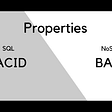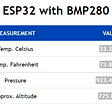# INTRODUCTION

Following article presents (2D and 3D) computed and plotted in C++.
Bézier curve has an excellent mathematical ability to compute path (curve from start to destination point). The shape of the curve is shaped by “control points”. The most the important feature of discussed curve is the .
There are several applications and domains, where the smoothness is indispensable. We can consider the motion of robot or other machine where movement have to be predictable, securing safety for human and hardware (low wear coefficient). When the the trajectory of robot joint is calculated as a smooth path, we can assume the robot is going to move according to planed path, smoothly without jerky or accidentally movements. Please note in robotics we consider, beside path — also velocity, acceleration, jerk and motor torque. All these parameters mainly influence for final path.
Beside robotics, Bézier curves are used in animation, games and design.

For plotting purpose I will use also discussed in my previous posts, the matplotlib library for C++.

The header file (for plotting library) has to be in the same folder as your cpp.
Your program can be compiled as follows,

`//compileg++ my_prog.cpp -o my_prog -I/usr/include/python3.8 -lpython3.8// //run./my_prog//folder tree├── my_prog├── my_prog.cpp├── matplotlibcpp.h`

# FEATURES

Bézier curve can be computed for set of points: {, where defines the order of curve (polynomial) we model. In each case the first and the last point defines the position of start and end of the curve. The other points — control points generally do not belong to the computed curve but rather influence the shape of the Bézier curve.

Each point in has the two Cartesian coordinates but in, the point is define as expected by three .

Explicit definition of the Bézier curve can be specified as follows (we will use this formula in our simulations).

Below equations are given in Latex format (here).

where

are the binomial coefficients.

In our case the binomial coefficients are computed as follows (if you check the Wikipedia you will find the recursive implementation, however this is easiest version or more intuitive).

The implementation in C++ can be as follows,

Four points 0, 1, 2 and 3 in the plane space define a cubic Bézier curve. The curve can be modeled as a polynomial of third order.

When six points 0, 1, 2, 3, P4 and P5 are provided so the Bézier curve is computed as a polynomial of fifth order.

# SIMULATIONS

Now we are going to display the 2D and 3D simulations of curves defined above (for 4 and 6 points). The code (below) give you an excellent possibility to compute and plot a Bézier curve for any number points you want.

Depicted below 2D Bézier curve, was computed for cubic polynomial (four points).

`x{2.5, 1.5, 6.0, 10.0};y{0.5, 5.0, 5.0, 0.5};`

For the same order of polynomial (third order) we can compute 3D Bézier curve.

`x{2.5, 1.5, 6.0, 10.0}; //same as 2Dy{0.5, 5.0, 5.0, 0.5}; //same as 2Dz{1.0, 2.0, 3.0, 4.0};`

Here is a 2D Bézier curve, which was computed for fifth order polynomial (six points).

`X{2.5, 1.5, 6, 10, 7, 3};Y{0.5, 5.0, 5.0, 0.5, 1.0 , 2.0};`

As before, we can plot 3D Bézier curve.

`X{2.5, 1.5, 6.0, 10.0, 7.0, 3.0}; //as for 2DY{0.5, 5.0, 5.0, 0.5, 1.0 , 2.0}; //as for 2DZ{1.0, 2.0, 3.0, 4.0, 5.0, 0.1};`

--

--

--

## More from Geek Culture

A new tech publication by Start it up (https://medium.com/swlh).

## Recommended from Medium## More from Medium